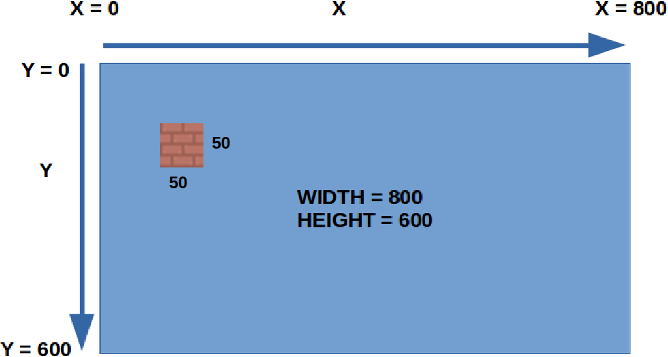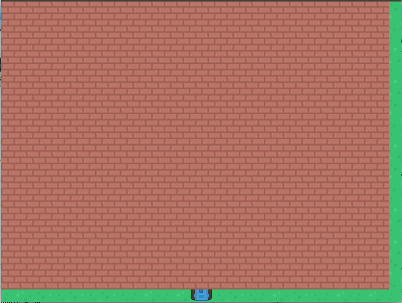# Walls

We'll start by filling up the entire screen with wall pieces. In the next section, we'll then remove some of the pieces randomly.

To create the many pieces of walls in the game, we will need to create a lot of actors. To help us, we will use a list and a for loop.

Each piece of wall is 50 pixels by 50 pixels, and the window size is 800 by 600...So how many pieces of the wall can fit horizontally? How about vertically?

### Horizontal

Width of screen = 800
Width of wall = 50
Number of wall pieces = 800 / 50 = 16 pieces

### Vertical

Height of screen = 600
Height of wall = 50
Number of wall pieces = 600 / 50 = 12 pieces

When drawing the wall, we need to repeat 16 times in the horizontal (x) direction, and 12 times in the vertical (y) direction.

``````walls = []
for x in range(16):
for y in range(12):
wall = Actor('wall')
wall.x = x * 50
wall.y = y * 50
walls.push(wall)
``````

This is what each line does...

`walls = []` Create an empty list (...no items in it).

`for x in range(16):`Repeat 16 times, setting the value to the variable x each time.

`for y in range(12):`Repeat 12 times, setting the value to the variable y each time.

`wall = Actor('wall')` We create an actor for one piece of wall.

`wall.x = x * 50` We multiply x by 50, because each wall section is 50 pixels wide.

`walls.push(wall)` We add the piece of wall into our list of walls.

### Drawing the wall

Just like the tank, we need to draw the wall in the draw() function. As we have a list of walls with many wall actors, we'll need to go through the list and draw each of them one by one using a for loop.

``````for wall in walls:
wall.draw()
``````

With that, your program should now look like this...

``````import pgzrun

WIDTH=800
HEIGHT=600

tank = Actor('tank_blue')
tank.y = 575
tank.x = 400
tank.angle = 90

background = Actor('grass')

walls = []
for x in range(16):
for y in range(12):
wall = Actor('wall')
wall.x = x * 50
wall.y = y * 50
walls.append(wall)

def update():
if keyboard.left:
tank.x = tank.x - 2
tank.angle = 180
elif keyboard.right:
tank.x = tank.x + 2
tank.angle = 0
elif keyboard.up:
tank.y = tank.y - 2
tank.angle = 90
elif keyboard.down:
tank.y = tank.y + 2
tank.angle = 270

def draw():
screen.fill((0,0,0))
background.draw()
tank.draw()
for wall in walls:
wall.draw()

pgzrun.go() # Must be last line
``````

### Fixing the wall

If you tried running the program, you'll see something like this...Remember that the position of an actor is based on its center. To fix this problem, we need to add half the width and height of the wall to its x and y. Since the width and height of the wall is 50, this means we need to add 25. Change the wall.x and wall.y lines to this...

``````wall.x = x * 50 + 25
wall.y = y * 50 + 25
``````

Done! The walls covered up our tank, but that's ok for now. In the next section, we'll remove some of the walls to make space for our tank.